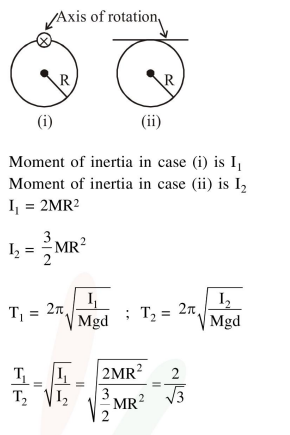Deepak Scored 45->99%ile with Bounce Back Crack Course. You can do it too!

# A ring is hung on a nail.

Question:

A ring is hung on a nail. It can oscillate, without slipping or sliding (i) in its plane with a time period $T_{1}$ and, (ii) back and forth in a direction perpendicular to its plane, with a period $T_{2}$. the ratio $\frac{\mathrm{T}_{1}}{\mathrm{~T}_{2}}$ will be :

1. $\frac{2}{\sqrt{3}}$

2. $\frac{\sqrt{2}}{3}$

3. $\frac{2}{3}$

4. $\frac{3}{\sqrt{2}}$

Correct Option: 1

Solution: